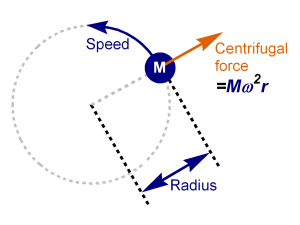# Centrifugal Force Calculator

Created by Luis Hoyos
Last updated: Jun 22, 2022

Rotational motion is present in many real-life situations, such as the movement of centrifugal pumps, car engines, orbital period formula, or the tagada of amusement parks; for that reason, we created this centrifugal force calculator.

In the following section, we talk about:

• The definition of centrifugal force.
• The acceleration and centrifugal force formulas.
• The equation for centrifugal force calculation, with velocity in rpm instead of rad/s.

🔎 If you're dealing with tangential forces, our angular displacement calculator can be helpful. Then, you can use that displacement and the tangential force to find the work using .

## Centrifugal force definition

Centrifugal force is the fictitious or inertial force that a rotating object feels. We consider it fictitious because it only appears in non-inertial frames of reference, while the observers in an inertial reference frame don't perceive it.

## Centrifugal acceleration formula

Understanding the centrifugal acceleration is necessary to know where the centrifugal force formula comes from.

The centrifugal acceleration formula is:

$a = \omega^2 r$

where:

• $a$ — centrifugal acceleration (m/s2);
• $\omega$ — angular speed (m/s); and
• $r$ — radial distance to the axis of rotation (m).

We can also express the centrifugal acceleration formula in terms of the tangential velocity $v$:

$a = \frac{v^2}{r}$

## Centrifugal force formula

The equation for centrifugal force comes from the definition of force, which says it equals mass times acceleration. Multiplying mass by angular acceleration, we have:

$F = m \omega^2r$

where:

• $F$ — Centrifugal force, usually expressed in newtons (check the for more information about force units);
• $m$ — Mass of the object;
• $\omega$ — Angular velocity
• $r$ — Radial distance to the axis of rotation.

As with centrifugal acceleration, we can express the centrifugal force equation in terms of tangential speed:

$F = \frac{m v^2}{r}$

Since the centrifugal force is strictly related to the rotational motion, you may want to express the coordinates with a more convenient system. Check our cartesian to polar coordinates calculator to see the latter method's advantages.

## Centrifugal force formula in rpm

Sometimes we need to calculate the centrifugal force, but we possess the angular velocity in rpm instead of rad/s. Instead of converting from rad/s to rpm, we can develop a centrifugal force formula for rpm inputs:

First, let's remember the relationship between rad/s to rpm.

$1\ \text{rad/s} = \frac{30}{\pi}\ \text{rev/min}$

Considering the previous relationship, we can calculate the centrifugal force (using rev/min) with the following formula:

$F = \frac{30}{\pi} m (\text{RPM})^2r$

In the previous formula, $RPM$ is the angular velocity in revolutions per minute.

Luis Hoyos
VelocitiesYou only need to add one type of velocity, either tangential or angular velocity.
Mass (M)
lb
ft
Tangential velocity (v)
ft/s
Angular velocity (ω)
rpm
Force and acceleration
Centrifugal force (F)
lbf
Centrifugal acceleration (a)
m/s²
People also viewed…

### Angular velocity

The angular velocity calculator offers two ways of calculating angular speed.

### Mass moment of inertia

Use this mass moment of inertia calculator to help you find the mass moment of inertia of an object or a point mass.